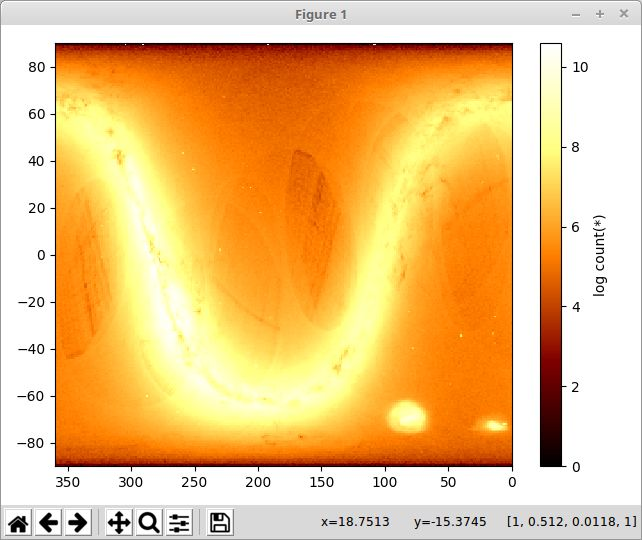# Vaex – Multi-code Analysis Toolkit for Visualization and Exploration of Big Tabular Data

Vaex is an open source program and Python library to visualize and explore large tabular datasets. It can calculate statistics such as mean, sum, count, standard deviation etc, on an N-dimensional grid up to a billion (109) objects/rows per second.

The original motivation to develop Vaex is the Gaia astronomical catalogue containing over a billion stars (at least for data release 1, DR1). Vaex can visualize the complete Gaia catalogue in one second.

Features:

• Graphical interface for most common uses cases.
• Visualize and explore big tabular data interactively
• Renders histograms, density plots and volume rendering plots for visualization in the order of a billion (109) objects in the order of 1 second.
• Explore the dataset by using visual queries and Boolean expressions to visualize subsets of the data.
• Statistics such as mean, sum, count, standard deviation etc, can all be calculated on an N-dimensional grid.
• For exploration it supports selection in 1 and 2d, but it can also analyze the columns (dimensions) to find subspaces which are richer in information than others.
• Overplot vectors, for instance mean motions, tensors (for instance mean velocity dispersion tensor).
• Custom expressions, e.g. log(sqrt(x**2+y**2)), calculated on the fly.
• Uses memory mapping, zero memory copy policy and lazy computations for best performance. Memory mapped files avoids unnecessary reading and copying of data. Binning or aggregating the data on a grid, using simple optimized algorithms. Columnar storage of data avoids reading unnecessary data and enables maximum performance of hard drives.
• Publish quality output (using matplotlib).
• Linked views: selecting in 1 view will also select it in different views.
• Data formats supported:
• hdf5 (Hierarchical Data Format): gadget, Vaex’s own format;
• hdf5 from Amuse;
• fits bintable;
• VOtable over SAMP;
• Client/server architecture: Delegate computations to a remote server.

The Vaex library generates the same plots and more, and offers integration with Jupyter/IPython notebook.

• pip and conda installable.
• Make custom plot and statistics.
• Calculate statistics on a N-dimensional grid and visualize it.
• Create interactive Jupyter/IPython notebooks.
• Publication quality plots with matplotlib.
• Interactive plots with bqplot or Bokeh.
• Combine the notebook with the graphical interface in one kernel.

Website: vaex.io
Support: Documentation, GitHub
Developer: Maarten A. Breddels